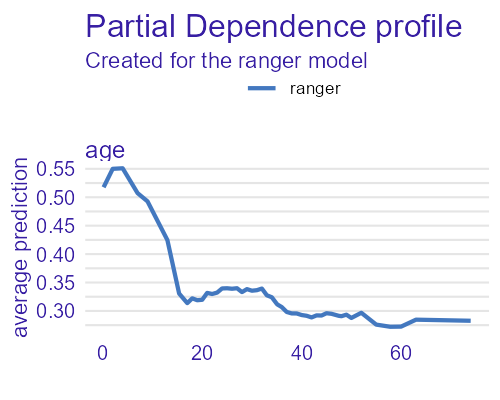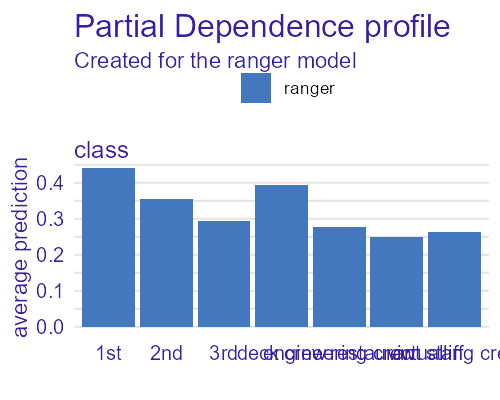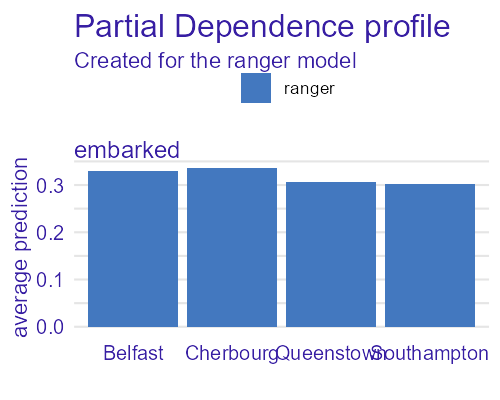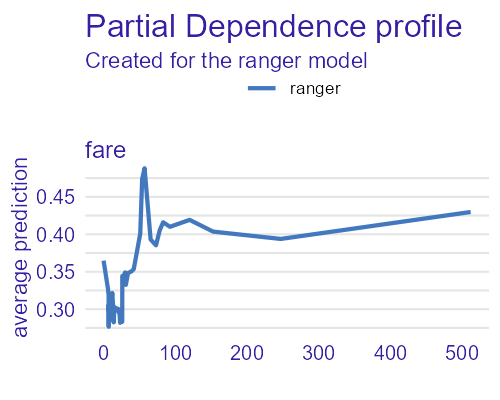## Custom correlation coefficients

### calculate_cors function

As mentioned in introduction, calculating comparable correlation coefficients between variables of different types is a non-trivial problem. The CorrGrapheR package provides user with ability to experiment with different measures of correlation, using calculate_cors function.

The num_num_f, num_cat_f and cat_cat_f arguments should be functions, used to calculate correlation coefficients between different pairs variables, respectively. Each of them should take 2 arguments (vectors of equal length; for num_num_f - both numeric, for cat_cat_f - both factor, for num_cat_f - first numeric and second factor) and return a single number. It should be interpretable in the following way: the bigger its absolute value, the stronger correlation between variables. If it’s negative - the variables are negatively correlated.

When supplying custom functions, a max_cor argument is required. It should be a single number indicating strongest possible correlation (like 1 in Pearson’s cor.test). It is used when calculating correlation between the same variables, to trim accidental results above it and to scale final results into $$[-1,1]$$ range.

The calculate_cors returns a correlation matrix, similarly to cor.

library(corrgrapher)
df <- as.data.frame(datasets::Seatbelts)[,1:5]
f1 <- function(x, y) cor.test(x, y, method = 'pearson')$estimate f2 <- function(x, y) cor.test(x, y, method = 'kendall')$estimate
f3 <- function(x, y) cor.test(x, y, method = 'spearman', exact = FALSE)$estimate calculate_cors(df, num_num_f = f1, max_cor = 1) #> DriversKilled drivers front rear kms #> DriversKilled 1.0000000 0.8888264 0.7067596 0.3533510 -0.3211016 #> drivers 0.8888264 1.0000000 0.8084114 0.3436685 -0.4447631 #> front 0.7067596 0.8084114 1.0000000 0.6202248 -0.3573823 #> rear 0.3533510 0.3436685 0.6202248 1.0000000 0.3330069 #> kms -0.3211016 -0.4447631 -0.3573823 0.3330069 1.0000000 calculate_cors(df, num_num_f = f2, max_cor = 1) #> DriversKilled drivers front rear kms #> DriversKilled 1.0000000 0.6785708 0.5085310 0.2602347 -0.1870987 #> drivers 0.6785708 1.0000000 0.6262218 0.2426445 -0.2895965 #> front 0.5085310 0.6262218 1.0000000 0.4518703 -0.2270979 #> rear 0.2602347 0.2426445 0.4518703 1.0000000 0.2135214 #> kms -0.1870987 -0.2895965 -0.2270979 0.2135214 1.0000000 calculate_cors(df, num_num_f = f3, max_cor = 1) #> DriversKilled drivers front rear kms #> DriversKilled 1.0000000 0.8579854 0.6936970 0.3835558 -0.2686338 #> drivers 0.8579854 1.0000000 0.8225164 0.3641043 -0.4234322 #> front 0.6936970 0.8225164 1.0000000 0.6086036 -0.3511428 #> rear 0.3835558 0.3641043 0.6086036 1.0000000 0.3140946 #> kms -0.2686338 -0.4234322 -0.3511428 0.3140946 1.0000000 As we see, user has to supply only necessary functions for given x argument. For a data.frame only with numeric data only num_num_f is required. Since correlation measures for different data variables are not comparable in most cases, it required from the user to always supply all necessary functions. Naturally, user might supply function identical to the default: data(dragons, package = 'DALEX') f1 <- function(x, y) -log10(cor.test(x, y, method = 'spearman', exact = FALSE)$p.value)
f2 <- function(x, y) -log10(kruskal.test(x, y)\$p.value)
calculate_cors(dragons,
num_num_f = f1,
num_cat_f = f2,
max_cor = 100)
#>                      year_of_birth       height       weight       scars
#> year_of_birth         1.0000000000 0.0040428398 1.084646e-02 0.005151935
#> height                0.0040428398 1.0000000000 1.000000e+00 0.001298727
#> weight                0.0108464600 1.0000000000 1.000000e+00 0.006402918
#> scars                 0.0051519347 0.0012987272 6.402918e-03 1.000000000
#> colour                0.0068233038 0.0004765201 4.282685e-05 0.003525012
#> year_of_discovery     0.0098803614 0.0030892743 2.337062e-03 0.040962737
#> number_of_lost_teeth  0.0007526137 0.0101897329 7.369186e-03 0.004277834
#> life_length           0.0104123400 0.0071877845 1.186213e-02 1.000000000
#>                            colour year_of_discovery number_of_lost_teeth
#> year_of_birth        6.823304e-03      0.0098803614         0.0007526137
#> height               4.765201e-04      0.0030892743         0.0101897329
#> weight               4.282685e-05      0.0023370625         0.0073691861
#> scars                3.525012e-03      0.0409627374         0.0042778339
#> colour               1.000000e+00      0.0060860485         0.0081442241
#> year_of_discovery    6.086049e-03      1.0000000000         0.0001690691
#> number_of_lost_teeth 8.144224e-03      0.0001690691         1.0000000000
#> life_length          3.046990e-03      0.0184173931         1.0000000000
#>                      life_length
#> year_of_birth        0.010412340
#> height               0.007187785
#> weight               0.011862132
#> scars                1.000000000
#> colour               0.003046990
#> year_of_discovery    0.018417393
#> number_of_lost_teeth 1.000000000
#> life_length          1.000000000

### Inside corrgrapher function

calculate_cors is called inside corrgrapher function. User may pass custom functions to it via cor_functions argument. It should be a named list with num_num_f, num_cat_f, cat_cat_f and max_cor elements.

corrgrapher(df,
cor_functions = list(num_num_f = f1,
num_cat_f = f2,
max_cor = 100))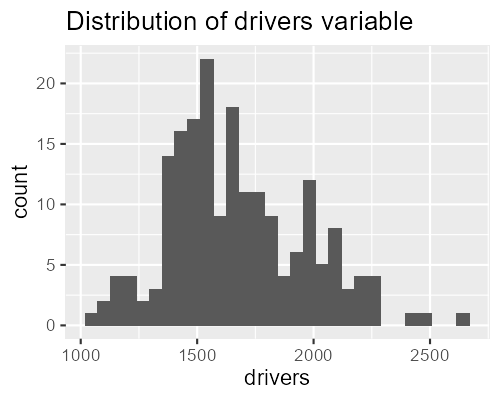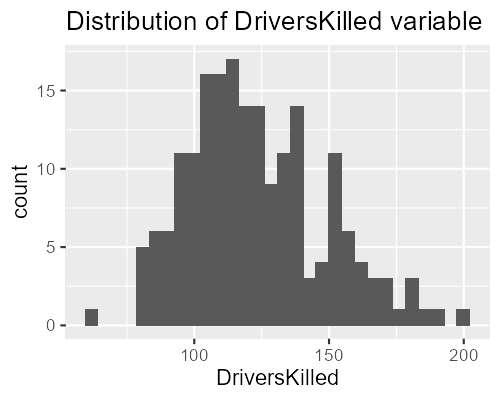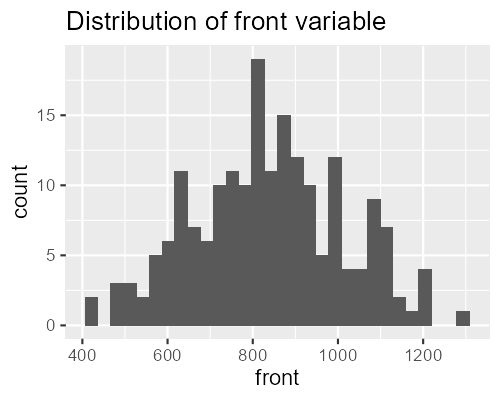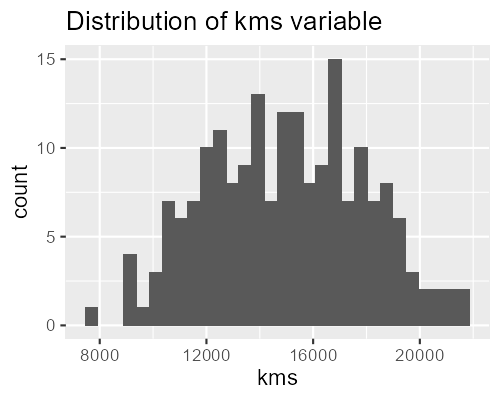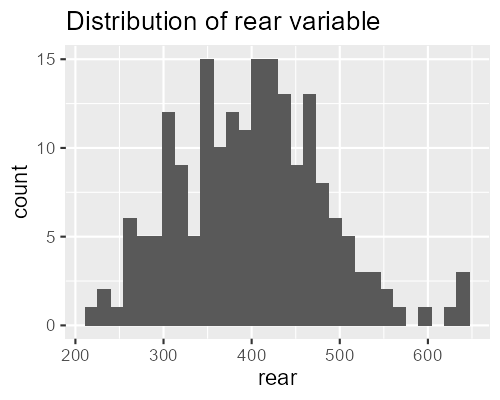## Custom feature importance

When corrgrapher function is called on an explainer object, it calculates inside importance of features (variables) using ingredients::feature_importance function. User may (sometimes should) supply feature_importance argument as either:

• An object created using ingredients::feature_importance on the same explainer, or
• A named list of parameters to pass to ingredients::feature_importance called inside corrgrapher function.

Remember, do not change variables and variable_groups arguments.

library(ranger)
library(DALEX)
#> Welcome to DALEX (version: 2.0).
#> Find examples and detailed introduction at: https://pbiecek.github.io/ema/
#> Additional features will be available after installation of: ggpubr.
#> Use 'install_dependencies()' to get all suggested dependencies
data("titanic_imputed", package='DALEX')
tit_model <- ranger(survived ~ ., data = titanic_imputed, num.trees = 100)
tit_model_exp <- explain(tit_model,
data = titanic_imputed[,-8],
y = titanic_imputed[, 8],
verbose = FALSE)
tit_model_fi <- ingredients::feature_importance(tit_model_exp,
B = 5,
loss_function = loss_accuracy)
tit_cgr_1 <- corrgrapher(tit_model_exp, feature_importance = tit_model_fi)
tit_cgr_2 <- corrgrapher(tit_model_exp,
feature_importance = list(B = 20,
loss_function = loss_one_minus_auc))
tit_cgr_1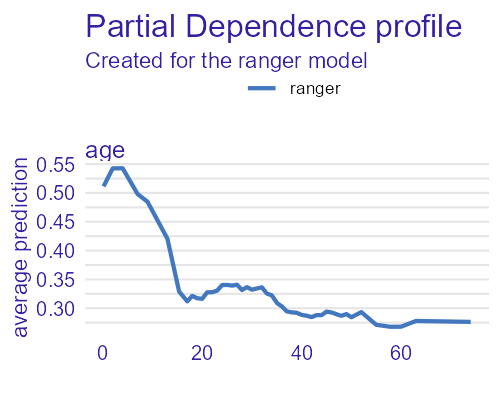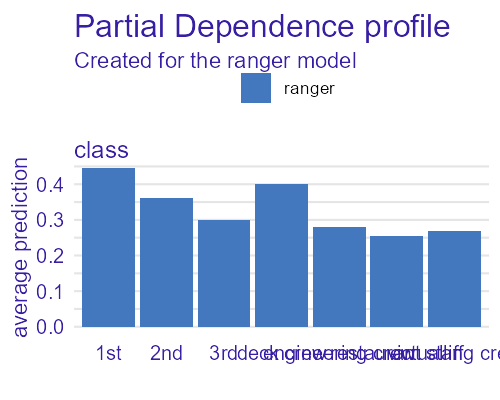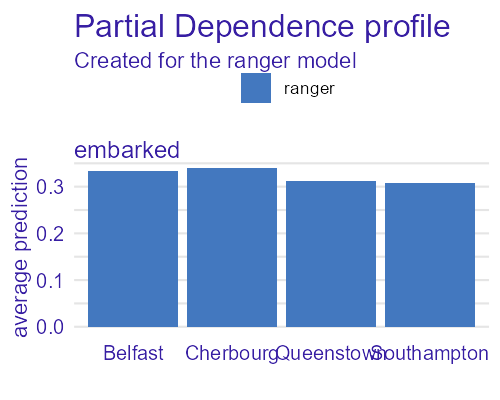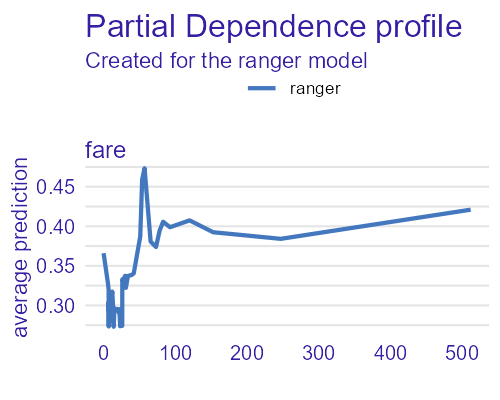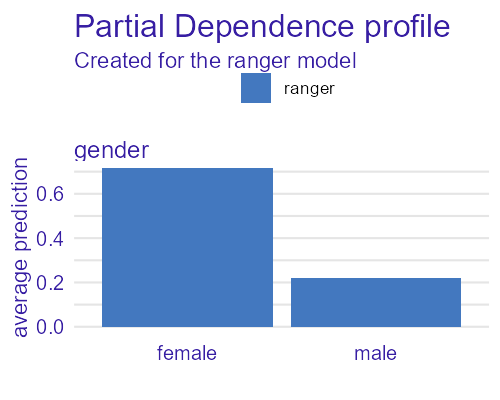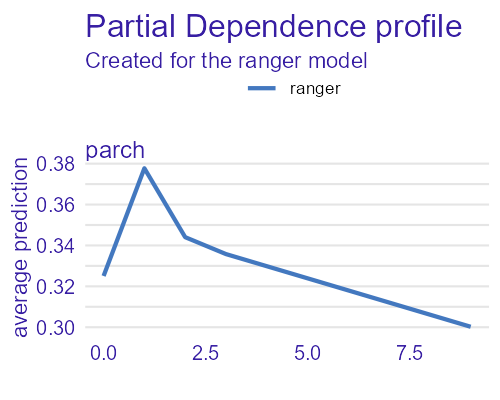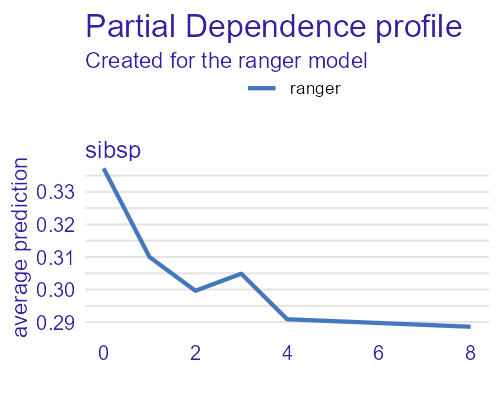tit_cgr_2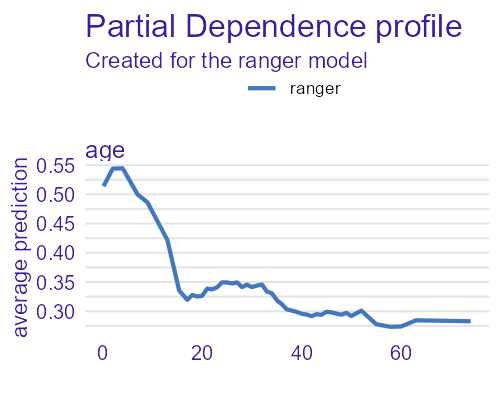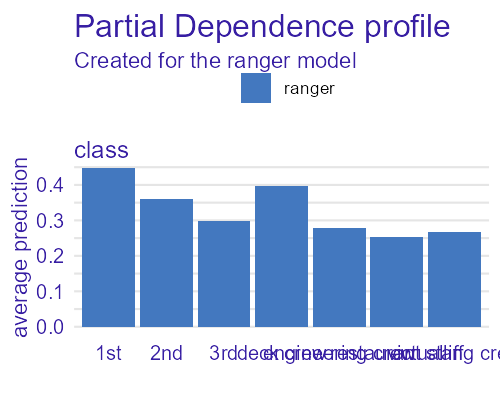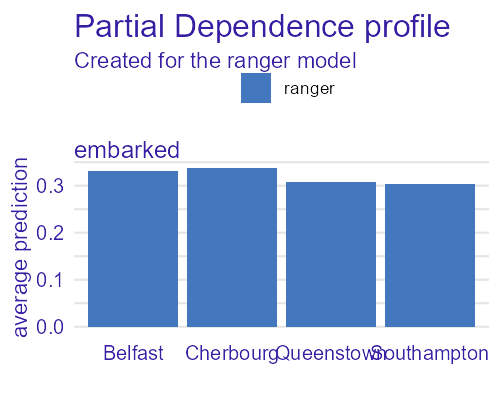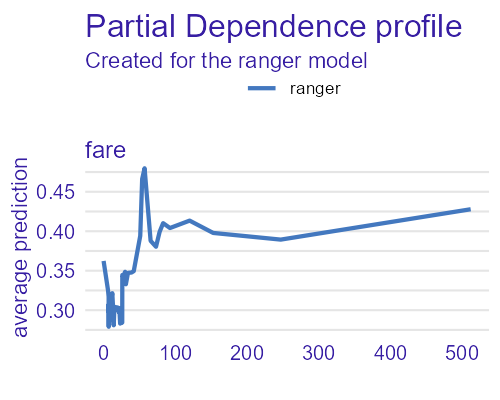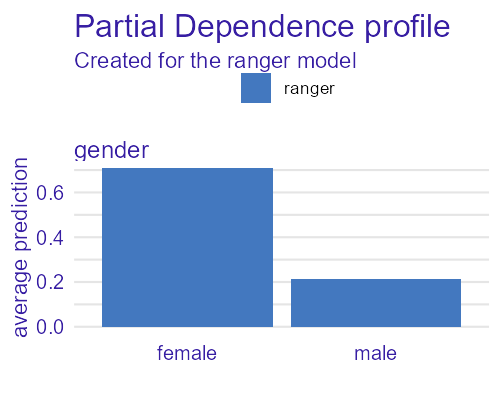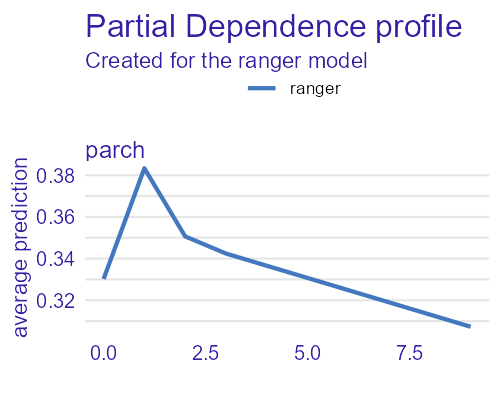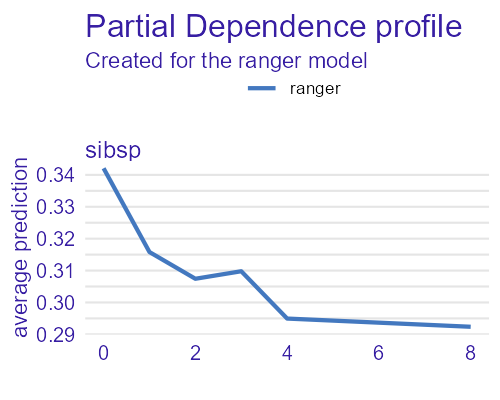## Custom partial dependence

Similarly to feature_importance, user may (sometimes should) supply partial_dependency argument. Do not change variable_type, variables or variable_splits arguments.

tit_model_pds <- ingredients::partial_dependence(tit_model_exp, grid_points = 50, N = 100)

tit_cgr_3 <- corrgrapher(tit_model_exp,
feature_importance = tit_model_fi,
partial_dependency = tit_model_pds)
tit_cgr_4 <- corrgrapher(tit_model_exp,
feature_importance = tit_model_fi,
partial_dependency = list(grid_points = 101,
N = 200))

tit_cgr_3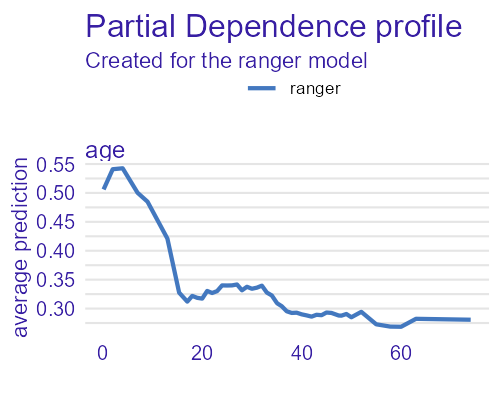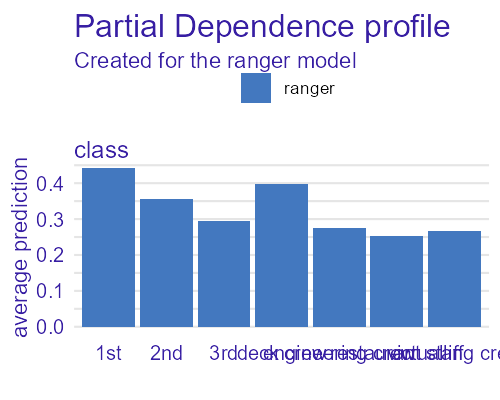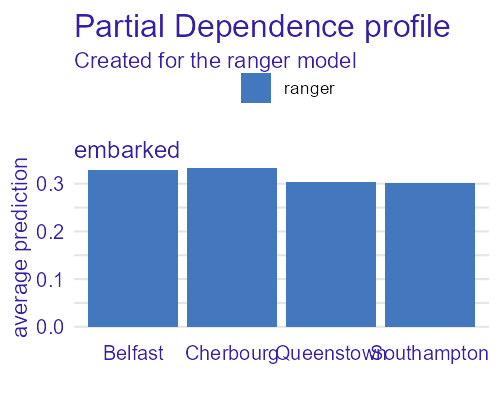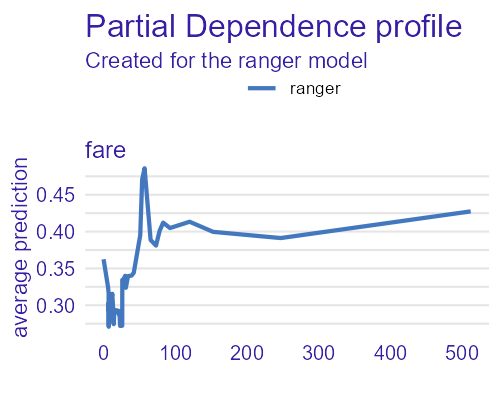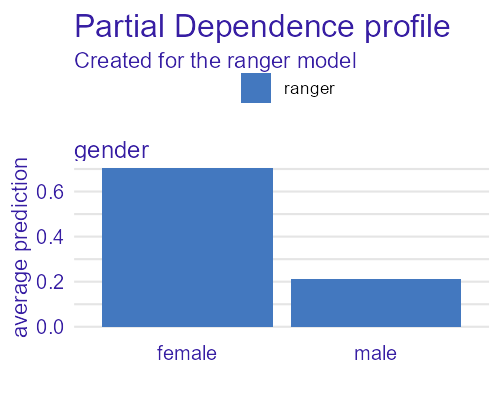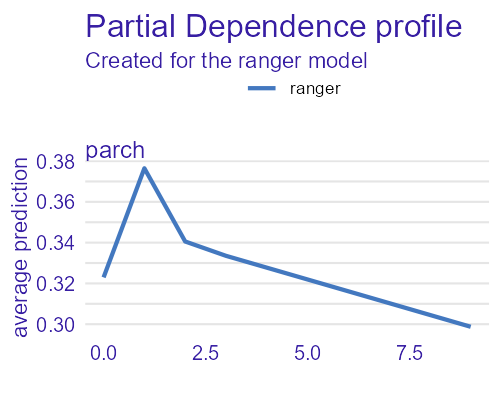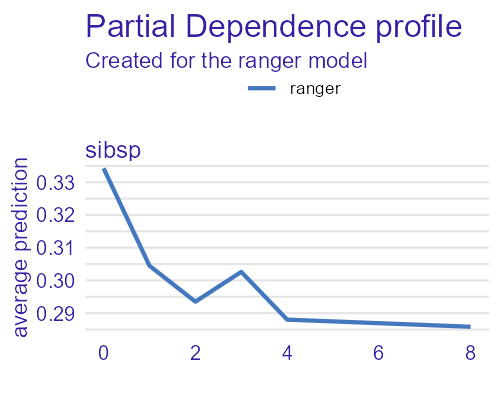tit_cgr_4